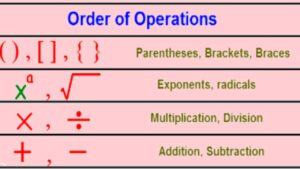October 7, 2022

Contents

# Order of Operations with Exponents

The rule to evaluate  the value of a mathematical expression that includes  exponents [e.g. (   -6 ÷ 3)  x 8 ], is  PEMDAS Stands for  P: Parentheses, E: Exponents ,  M:Multiplication , D: Division,  A: Addition and S: Subtraction.What do you do first in order of operations ?

The following steps must be followed to solve a mathematical expression that includes an exponents:

Step 1: Operations inside the parentheses should be solved first .

Step 2:  After performing operations inside of parenthesis , solve the exponents .

Step 3:  After the parentheses  and the exponents, perform multiplication or division from left to right depending on which operation comes first.

Step 4:  Finally, after multiplication and division,  perform addition and subtraction from left to right depending on which operation comes first.

## Order of Operations with Exponents(Solved examples)

(i) (9+43-4) -8-

Sol. (9+43-4) -8-=  48-8-         (Performing the operations inside the parentheses)

= 48-8-9             (solving exponent)

=31                       (performing subtraction)

(ii)  33+10-÷

Sol. 33+10-÷= 33+10-16÷                            (performing exponents)

=33+10-2                                        (performing division)

=33+10-62                                             (performing multiplication)

= 43-62                                                     (performing addition)

= -19                                                           (performing subtraction)

(iii)  70÷7-

Sol. 70÷7-=  70÷7-27                      (performing exponent)

=10-27                               (performing division)

=-17                                     (solving subtraction)

(iv) ÷ 4

Sol. ÷ 4 = (1 x 4+27) -32 ÷ 4

=( 4+27) -32 ÷ 4

=31-32  ÷ 4

= 31- 8

= 23

(v)  25-8 x 2 +

Sol. 25-8 x 2 + =25-8 x 2 +9

= 25-16 +9

=9+9

=18

(vi) ÷ 4

Sol.  ÷ 4 =÷ 4

= 81  +3 x 16÷ 4

=81+48 ÷ 4

=81+12

=93

(vii) 36÷

Sol.  36÷= 36÷[20-8]                     (solving the operations inside the parentheses)

=36 ÷12

=36÷12+16-6                                 (solving exponent)

=3+16-6                                            (performing division)

=13

(viii)

Sol. =

=

=50-2+16

= 48+16

= 64

(ix) ( ÷ 15)

Sol. (÷ 15) =(3 x 25 ÷ 15) -(5-4)

=(75 ÷15)-(5-4)

= 5-4

=1

(x) ÷

Sol.   ÷   =   2 x 6 ÷ 6 – +3 x 4

=  2 x 6 ÷ 6 – 8 +3 x 4

= 12 ÷ 6 – 8 +3 x 4

=2 – 8 +3 x 4

=2-8+12

=-6+12

=6

### Order of Operations with Exponents  (Unsolved examples with answers)

(i)  (   -6 ÷ 3)  x 8

(ii)

(iii)

(iv)

(v) (22÷ 11)

(vi) 5+21÷

(vii)

(viii) ÷ 4

(ix) 12 ÷4

(x)

(xi) 33+10-÷

(xii) ÷   +14 x 3

(xiii) ÷

(xiv)   ÷5-11

(xv) ÷ 9

Ans.  (i) 56    (ii) 126    (iii) -13   (iv) 95   (v) -60   (vi ) 0   (vii) 29  (viii) -5  (ix) 67  (x) 58  (xi) -19   (xii) 75   (xiii) 13

(xiv)  126    (xv) 13

#### Order of Operations with Exponents worksheet PDF with answers

Download PDF  order of operations with exponents

You might also be interested in:#### Bina singh

View all posts by Bina singh →
List of Google Scholarships IBPS Clerk 2022 Mains Exam Admit card 2022 CUET-PG results Scheduled Exams in October 2022 SBI Notification for the 1673 Probationary Officers